# A Research on Selection of Appropriate Stability Index under Adverse System Conditions for the Assessment of Voltage Stability of an IEEE 14 Bus Power System

A Research on Selection of Appropriate Stability Index under Adverse System Conditions for the Assessment of Voltage Stability of an IEEE 14 Bus Power System
Department of Electrical Engineering, National Institute of Technology Silchar, Silchar 788010, Assam, India

Corresponding Author Email:
pawan_rs@ee.nits.ac.in
Page:
435-446
|
DOI:
https://doi.org/10.18280/ejee.220605
29 June 2020
|
Accepted:
10 October 2020
|
Published:
31 December 2020
| Citation

OPEN ACCESS

Abstract:

For voltage stability assessment at a given operating point, various types of voltage stability indices (VSIs) have been proposed in the literature. In this paper, the voltage stability assessment of an IEEE-14 bus system is done for performance comparison of different types of VSIs available, under certain critical and practical stressed operating conditions (SOCs). The performance comparison of various VSIs under the considered SOCs is not reported in the literature. Such SOCs include the combinational occurrence of – variation in inductive loading, single line to ground (SLG) fault and effect of one generation unit tripped. These SOCs are the prime cause of voltage collapse of any node/line. The results show the performance of various VSIs with respect to line number, contingency ranking of the line, power margin, effects of loading and SLG fault. These VSIs are also instrumental in critical line and node analysis (CLNA) which is useful in the choice of proper location for reactive power compensation required. The simulated results provide the best performing VSI for accurate prediction of voltage instability under any considered SOC. This information is essential for voltage stability assessment of a particular line under multiple causes of voltage collapse.

Keywords:

contingency analysis, one generation unit tripped, power margin analysis, single line to ground fault, voltage stability indices and SLG fault

1. Introduction

The restrictions due to environmental conditions such as climatic effect, change in landscape, restriction on land use, impact on nature, self-regulation approach of transmission and distribution companies and excessive assembling price of new power lines, enforce the prevailing electricity structure to function under the burdened situation. Any perturbation in this situation may cause voltage instability. Consequently, the investigation of voltage stability is vital for a power system. The dynamic and static approach of voltage stability is often used for the analysis of stable voltage points in a power system. More computational time is required for dynamic stability analysis compared to the static approach . The stability of a power system using real power versus line voltage (PV) and reactive power versus line voltage (QV) curves are analyzed but these techniques are time-consuming. Therefore, the methods based on voltage stability indices (VSIs) are used for faster voltage stability estimation. A numeric value of VSIs specifies the voltage stability of the system. In the literature various types of VSIs are proposed under general system conditions like base loading, single continuous real load change, single continuous reactive load change and multiple real load change but correct choice of a VSI depends on the more severe stressed operating conditions of the power system. So for an appropriate selection of VSI, evaluation of its numeric value along with different performance analysis is essential. This is because different VSI may be appropriate under different SOCs and stability analysis for a particular line/node. A Fast Voltage Stability Index (FVSI) is proposed by Musirin and Rahman  for the prediction of the point of voltage collapse, maximum acceptable load, feeble bus in the system and the critical lines with respect to voltage stability in the system. But FVSI gives inaccurate result in case of real power increment, as it neglects the real power flow in the line. Moghavvemi and Omar  proposed the Line Stability Index (Lmn) which depends on the reactive power. Due to its negligence of real power flow and the consideration of shunt admittance to be approximately zero it gives less magnitude of Lmn while the line voltage is closer to its unstable point.

Thus from the above literature, the effectiveness of the VSIs has been investigated on a standard IEEE-14 bus system under stressed operating conditions (SOCs) like

2. Single line to ground (SLG) fault.

3. One generation unit tripped (OGT).

The remaining paper is organized as follows: Section 2 represents mathematical expressions of indices. Problem formulation is discussed in Section 3. The simulation results and their discussion are presented in Section 4 and in Section 5 conclusion is drawn.

2. Voltage Stability Indices

The line VSIs for a two-bus system as represented in Figure 1, are formulated in literature by considering the assumptions. The mathematical expressions of different VSIs obtained from the literature with their assumption and their critical values are listed in Table 1. These expressions are utilized for the calculation of their values in different system conditions. After computation and comparison of VSIs with their critical value for a line, the voltage stability of that line can be ascertained.

## 1.png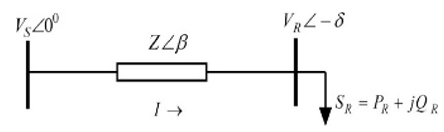Figure 1. Two bus system supplying load through the transmission line 

3. Problem Formulation

The notation and meaning of different variables which are used in the Table 1 are as follows – sending end and receiving end voltages (VS, VR), sending end and receiving end active powers (PS, PR), sending end and receiving end reactive powers (QS, QR), power angle (δ), line resistance (R), line reactance (X), line impedance (Z), line susceptance (B), phase angle of parameter A (α), phase angle of parameter B (β), impedance angle of line (θ) and voltage difference between sending and receiving end voltages (∆V).

## 2a.png(a) IEEE-14 Bus System 

## 2b.png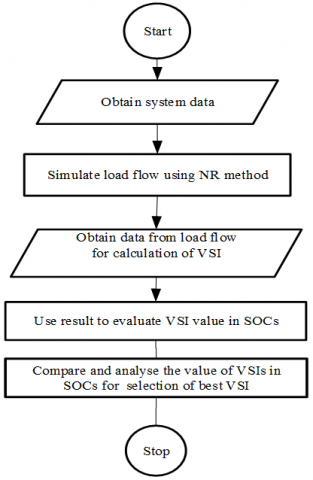(b) Flow chart of the proposed method

Figure 2. Single line diagram of system and flow chart of the proposed method

Table 1. Expressions for assumed VSI with critical values

 S. No Ref. VSI CV Mathematical Expressions Assumption 1 1 FVSI 1 $F V S I=\frac{4 Z^{2} Q_{R}}{V_{S}^{2} X}$ sin δ≈0, cos δ≈1, R sin δ≈0, X cos δ≈X 2 2 Lmn 1 $L_{m n}=\frac{4 X Q_{R}}{\left(V_{S} \sin (\theta-\delta)\right)^{2}}$ Y≈0, Effect of active power neglected 3 3 LQP 1 $L Q P=4\left(\frac{X}{V_{S}^{2}}\right)\left(Q_{R}+\frac{P_{S}^{2} X}{V_{S}^{2}}\right)$ Y≈0, R≈0 4 4 LP 1 $L_{P}=\frac{4 R P_{R}}{\left(V_{S} \cos (\theta-\delta)\right)^{2}}$ Y≈0, Effect of reactive power neglected 5 5 NLSI 1 $N L S I=\frac{P_{R} R+Q_{R} X}{0.25 V_{S}^{2}}$ Y≈0, δ≈0 6 6 VCPI 1 $V C P I(1)=\frac{P_{R}}{P_{R(\max )}}$ Constant power factor, Y≈0 7 7 NVSI 1 $N V S I=\frac{2 X \sqrt{P_{R}^{2}+Q_{R}^{2}}}{2 Q_{R} X-V_{S}^{2}}$ Y≈0, R≈0 8 8 VQILine 1 $V Q I_{\text {Line }}=\frac{4 Q_{R}}{|B| V_{S}^{2}}$ Y≈0, δ≈0 9 9 PTSI 1 $P T S I=\frac{2 S_{R} Z(1+\cos (\theta-\emptyset)}{V_{S}^{2}}$ Y≈0 10 10 VSI_1 0 $V S I_{-} 1=\min \left(\frac{P_{m \arg i n}}{P_{\max }}, \frac{Q_{m \arg i n}}{Q_{\max }}, \frac{S_{m \arg i n}}{S_{\max }}\right)$ Y≈0, R≈0 11 11 VSLI 1 $V S L I=\frac{4\left[V_{S} V_{R} \cos (\delta)-V_{R}^{2} \cos (\delta)\right]}{V_{S}^{2}}$ Y≈0 12 13 VSMs 0 $V S M_{S}=\frac{S_{C R}-S_{L}}{S_{C R}}$ Constant power factor, Y≈0 13 14 VCPI_1 0 $V C P I_{-} 1=V_{R} \cos (\delta)-0.5 V_{S}$ Zth(s)≈0 14 15 VSI_2 1 $V S I_{-} 2=\frac{4 Q_{R}(R+X)^{2}}{X\left(V_{S}^{2}+8 R Q_{R}\right)}$ Y≈0, δ≈0 15 16 VSMI 0 $V S L B I=\frac{V_{R}}{\Delta V}$ Y≈0, R≈0 16 17 VSLBI 1 $V S L B I=\frac{V_{R}}{\Delta V}$ Zth(s)≈0 17 18 SI 0 $S I=2 V_{S}^{2} V_{R}^{2}-V_{R}^{4}-2 V_{R}^{2}\left(P_{R} R+Q_{R} X\right)-Z^{2}\left(P_{R}^{2}+Q_{R}^{2}\right)$ Y≈0 18 19 LCPI 1 $L C P I=\frac{4|A| \cos (\alpha)\left[P_{R}|B| \cos (\beta)+Q_{R}|B| \sin (\beta)\right]}{\left[V_{S} \cos (\delta)\right]^{2}}$ Lines are considered as pie modeled 19 20 LVSI 1 $(L V S I)_{j}=\frac{2 V_{R j} A_{j} \cos \left(\beta_{j}-\alpha_{j}\right)}{V_{S j} \cos \left(\beta_{j}-\delta_{S R j}\right)}$

4. Simulation Results and Discussions

In this study, bus no 1 is considered as slack bus and as proposed in the IEEE 14 bus system as shown in Figure 2a, whereas, the buses 2, 3, 6, and 8 are considered as PV buses and remaining buses as load buses. The system consists of 20 interconnected lines. Different VSIs are calculated using the data collected from load flow. The test results for different SOCs are presented in the following subsections under this section. VSIs for all the following general loading conditions, as obtained from the literature, are also computed but comparative simulated analyses are done only on the SOCs which are the extended effort of this work. The following are the general loading conditions.

2. Single continuous real load change only

3. Single continuous reactive load change only

4. Single continuous real and reactive load change

5. Single maximum real load change only

6. Single maximum reactive load change only

7. Single maximum real and reactive load change

8. Multiple real load change only

9. Multiple reactive load change only

10. Multiple real and reactive load change

Only the considered SOCs are discussed in detail in the following subsections. For the selection of an appropriate VSI in a particular case, first, we should decide the most critical line based on voltage magnitude, active and reactive power margins. The line which has less voltage magnitude and power margin known by the data obtained from load flow analysis. After deciding the most critical line, Table 3 needs to be followed for selecting the most performing VSI in a particular SOC.

4.1 IEEE-14 bus system with an SLG fault in different lines

It is well known that in overhead transmission and distribution of electric power, the probability of fault occurrence is more than underground power dispatch. Type of faults that may occur is a single line to ground fault (SLG), double line to the ground fault, line to line fault and three-line to the ground fault. But due to its highest probability of occurrence, SLG fault is only considered in this work. The following four combinations of loading with SLG fault are considered

• SLG fault in different lines one at a time under base case loading

• SLG fault in different lines one at a time under single maximum real loading

• SLG fault in different lines one at a time under single maximum reactive loading

• SLG fault in different lines one at a time under single maximum real and reactive loading

As the last case considered is the most critical above the others in terms of voltage instability, VSI performance is tested for this case only as shown in Figure 3. For any of the other cases, the best VSI may be followed. Figure 3 also shows how the most performing VSI (VSLI) performs for all the lines in the considered system. The best performing VSI is shown as a line chart on the secondary axis. This graphical representation is followed for all the remaining figures representing the best VSI performances.

4.1.1 System for single maximum real and reactive loads with an SLG fault in different lines tested one at a time

When both real and reactive single maximum load changes are considered with different SLG fault location, the range of variation in VSI magnitude is more than only single maximum real load change and less than only single maximum reactive load change as shown in Figure 3. The authors in the studies [11, 18-20, 22] have considered different loading conditions only without any line fault whereas in this work single maximum real and reactive loads with SLG fault in different lines are considered. Further, comparative analysis among various VSIs based on changes in their magnitude is demonstrated as in Figure 3. In this considered case best VSI is found VSLI and shown in the secondary axis in Figure 3.

4.2 System with one reactive power generation unit tripped

Here, one reactive power generation unit is considered absent along with different loading conditions, separately and also with mixed loading conditions. It is considered that node 8 of the IEEE 14-bus system shown in Figure 2a, supply no reactive power. This analysis helps us to know the capability of VSI to predict the voltage stability of the power system. The following combinations of loading are considered.

• Single maximum real and reactive load

• Multiple real and reactive load

• Mix case of the above combination

All the above cases are analyzed as done in section. 4.1. Results of the most critical case, which is the last case considered, in terms of voltage stability is demonstrated in Figure 4. This is discussed in the following subsection under this section. For any other cases, where the voltage degradation is less, in any line than this very case, the best performing VSI of the most critical case may be followed.

4.2.1 System with mixed loading case and one reactive power generation unit tripped

Critical line and node analysis (CLNA) are discussed by Tiwari et al.  but a specific emphasis on it regarding voltage instability is not discovered from the literature. In a power system, CLNA is crucial in deciding on a new load connection, an extension of the power line, location of reactive power compensation and an overall improvement in power quality. Following general loading conditions are considered which are obtained from literature.

All the above conditions are considered for testing voltage stability of lines but only results of critical conditions in which more voltage instability occurred are shown in the following subsections under this section.

Table 3. Selection of appropriate VSI

## 3.pngFigure 3. Graphical representation of VSIs in case of single maximum real and reactive loads with an SLG fault in different lines tested one at a time

## 4.png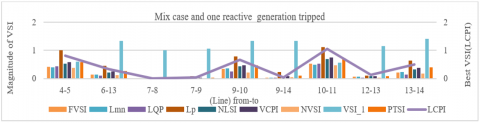Figure 4. Graphical representation of VSIs in case of mixed loading case and one reactive power generation unit tripped

## 5a.png(a) Graphical representation of VSIs with CLNA in single maximum real and reactive loading

## 5b.png(b) Graphical representation of VSIs with CLNA in multiple real and reactive load changes at the different load bus

## 5c.png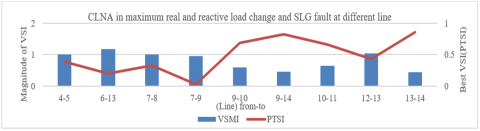(c) Graphical representation of VSIs with CLNA in maximum real and reactive load change and SLG fault at different line

## 5d.png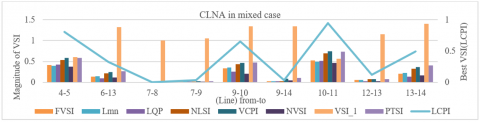Figure 5. Graphical representation of VSIs with CLNA in various loading case

## 6a.png(a) Graphical representation of VSIs with CA in pre-specified real and reactive loading

## 6b.png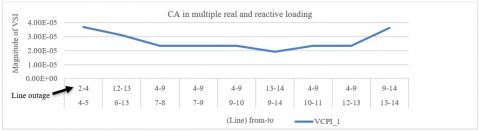(b) Graphical representation of VSIs with CA in multiple real and reactive loading

## 6c.png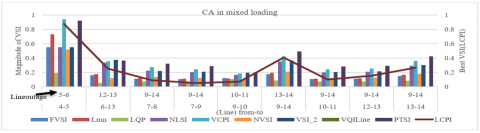Figure 6. Graphical representation of VSIs with CA in various loading case

4.3 System with CLNA

Critical line and node analysis (CLNA) are discussed by Tiwari et al.  but a specific emphasis on it regarding voltage instability is not discovered from the literature. In a power system, CLNA is crucial in deciding on a new load connection, an extension of the power line, location of reactive power compensation and an overall improvement in power quality. Following general loading conditions are considered which are obtained from literature.

All the above conditions are considered for testing voltage stability of lines but only results of critical conditions in which more voltage instability occurred are shown in the following subsections under this section.

According to the cases considered in Section 4.3.1- 4.3.4, the values of VSI for most stressed lines and nodes are analyzed under different loading conditions. Figure 5a represents the variation in VSI magnitude for different lines. The change in magnitude and the superiority of LQP is shown in Figure 5a on the secondary axis. Further, this work studies single maximum real and reactive loading with CLNA which is a novel contribution of this work not discussed in the studies [1-16, 21-23]. Next, LQP is compared with other VSIs as shown in Figure 5a.

4.3.2 System with CLNA in multiple real and reactive load changes at the different load bus

Figure 5b represents the variation of VSIs magnitude for a different line. The simulated result validates VCPI_1 dominance and variation as plotted in Figure 5b on the secondary axis. Moreover, this work put efforts to study with CLNA in multiple real and reactive load changes at the different load bus, compare with other VSIs which is a novel contribution of this work not discussed in the studies [1-16, 21-23].

4.3.3 System with CLNA in maximum real and reactive load change and SLG fault at different line

Figure 5c represents the variation of VSIs magnitude for a different line. The simulated result validates the PTSI dominance and Variation as plotted in Figure 5c on the secondary axis. Additionally, this work put efforts to study with CLNA in maximum real and reactive load change and SLG fault at different lines, compare with other VSIs which is a novel contribution of this work not discussed in the studies [1-16, 21-23].

Figure 5d represents the variation of VSIs magnitude for a different line. The simulated result validates the LCPI dominance and Variation as plotted in Figure 5d on the secondary axis. Additionally, this work put efforts to study with CLNA in mixed loading cases at different lines, compare with other VSIs which is a novel contribution of this work not discussed in the studies [1-16, 21-23].

4.4 System with CA

As found from literature, that single line contingency analysis (CA) is done under base case loading only [19, 20] by removing one line at a time. From the solution of power flow study, all the VSIs are evaluated. For this analysis, four loading combinations are considered out of which three new loading cases with CA are considered as the novel work is done.

The effects of all the aforementioned loading cases are analyzed but only the results of novel loading cases which are more harmful in terms of voltage degradation, are described in the following subsections.

A condition of line outages with the respective stressed line is analyzed in this section under prespecified real and reactive loading (section 3.1.7). A similar type of analysis is done for multiple real and reactive loadings and for the mixed loading case. In this case, at node 6 prespecified real and reactive powers with value 1.74 P.U are considered. Figure 6a represents the variation of VSIs for different lines of the IEEE 14 bus system. The result also confirms the superiority and change in the magnitude of VSLI shown on the secondary axis in Figure 6a.

In this particular case, multiple real and reactive loads are considered with loading multiplier for which load flow is converged such as λ= 1.52 P.U at nodes 4, 5, 6, 9, 10, 11, 12, 13 and 14 of the considered system. Figure 6b represents the variation of VSIs for different lines. The simulated result validates VCPI_1 dominance and variation as plotted in Figure 6b on the secondary axis.

In the mixed loading case, load at node 6 is 1.74 P.U pre-specified loads are considered, with loading multiplier λ= 1.52 P.U at nodes 4, 5, 9, 10, 11, 12, 13 and 14 loads considered and the rest of the load are set at base case load. Figure 6c represents the variation of VSIs for different lines. In this considered case best VSI is found LCPI and shown in the secondary axis in Figure 6c.

4.5 System with PMA

Power margin analysis (PMA) of a line is done by different VSI for different loading conditions. By adding more loading cases the capability of power margin prediction by VSI is explored. The following are the general loading conditions considered (4).

• Single continuous real load change only

• Single continuous reactive load change only

• Single continuous real and reactive load change

• Single maximum real load change only

• Single maximum reactive load change only

• Single maximum real and reactive load change

• Multiple real load change only

• Multiple reactive load change only

• Multiple real and reactive load change

We go through all subcases but only simulated results of PMA in single continuous real and reactive loading are shown in the following subsections under this section. Other subcases are analyzed in a similar manner.

In this case, a single maximum real and reactive load changes at the different lines are considered and VSIs for those lines are calculated. For different values of VSI, the power margin is calculated as per :

(1-VSIM)×MPM{when VSI value is less than 0.5}    (1)

(VSIM) × MPM {when VSI value is greater than 0.5}    (2)

## 7.pngFigure 7. Graphical representation of VSIs with PMA in single continuous real and reactive loading

In Eqns. (1) and (2), VSIM stands for VSI magnitude and MPM stands for maximum power margin. A similar type of analysis is done for the other cases with only the loading conditions being changed as discussed in section 4.5. In Figure 7, APM stands for actual power margin and EPM stands for evaluated power margin. This figure represents the variation of power margin by VSI magnitudes for different lines. In this considered case best VSI is found VCPI_1 and shown in the secondary axis in Figure 7.

In section 4.3.2, 4.4.2 and 4.5.1 the best performing VSI is VCPI_1 because real and reactive load change considers with multiple, single maximum, CLNA, CA, and PMA. The best performing VSI is found LCPI in section 4.2.1, 4.3.4, and 4.4.3 due to the consideration of mixed loading cases with OGT, CLNA, and CA. Section 4.1.1 and 4.4.1 shows that the VSLI is best performing VSI as real and reactive load change with single maximum, pre-specified, SLG and CA. After discussing all the cases it is observed that the best performing VSI is not changed if the base loading case is not changed and other auxiliary cases like CLNA, CA, PMA, OGT, and SLG are varying.

5. Conclusion

References

 Musirin, I., Rahman, T.A. (2002). Novel fast voltage stability index (FVSI) for voltage stability analysis in power transmission system. Student Conference on Research and Development, Shah Alam, Malaysia, pp. 265-268. https://doi.org/10.1109/SCORED.2002.1033108

 Moghavvemi, M., Omar, F.M. (1998). Technique for contingency monitoring and voltage collapse prediction. IEE Proceedings-Generation, Transmission and Distribution, 145(6): 634-640. https://doi.org/10.1049/ip-gtd:19982355

 Chebbo, A.M., Irving, M.R., Sterling, M.J.H. (1992). Voltage collapse proximity indicator: Behaviour and implications. In IEE Proceedings C (Generation, Transmission and Distribution), 139(3): 241-252. https://doi.org/10.1049/ip-c.1992.0037

 Moghavvemi, M., Faruque, M.O. (2001). Technique for assessment of voltage stability in ill-conditioned radial distribution network. IEEE Power Engineering Review, 21(1): 58-60. https://doi.org/10.1109/39.893345

 Yazdanpanah-Goharrizi, A., Asghari, R. (2007). A novel line stability index (NLSI) for voltage stability assessment of power systems. In Proceedings 7th WSEAS Int. Conf. on Power Systems, pp. 164-167.

 Moghavvemi, M., Faruque, O. (1998). Real-time contingency evaluation and ranking technique. IEE Proceedings-Generation, Transmission and Distribution, 145(5): 517-524. https://doi.org/10.1049/ip-gtd:19982179

 Kanimozhi, R., Selvi, K. (2013). A novel line stability index for voltage stability analysis and contingency ranking in power system using fuzzy based load flow. Journal of Electrical Engineering & Technology, 8(4): 694-703. https://doi.org/10.5370/JEET.2013.8.4.694

 Althowibi, F.A., Mustafa, M.W. (2010). Line voltage stability calculations in power systems. 2010 IEEE International Conference on Power and Energy, Kuala Lumpur, pp. 396-401. https://doi.org/10.1109/PECON.2010.5697616

 Nizam, M., Mohamed, A., Hussain, A. (2006). Dynamic voltage collapse prediction in power systems using power transfer stability index. 2006 IEEE International Power and Energy Conference, Putra Jaya, pp. 246-250. https://doi.org/10.1109/PECON.2006.346655

 Gong, Y., Schulz, N., Guzman, A. (2006). Synchrophasor-based real-time voltage stability index. 2006 IEEE PES Power Systems Conference and Exposition, Atlanta, GA, pp. 1029-1036. https://doi.org/10.1109/PSCE.2006.296452

 Makasa, K.J., Venayagamoorthy, G.K. (2011). On-line voltage stability load index estimation based on PMU measurements. 2011 IEEE Power and Energy Society General Meeting, Detroit, MI, USA, pp. 1-6. https://doi.org/10.1109/PES.2011.6039882

 Maharjan, R., Kamalasadan, S. (2015). Voltage stability index for online voltage stability assessment. 2015 North American Power Symposium (NAPS), Charlotte, NC, pp. 1-6. https://doi.org/10.1109/NAPS.2015.7335245

 Deng, P., Sun, Y., Xu, J. (2009). A new index of voltage stability considering distribution network. 2009 Asia-Pacific Power and Energy Engineering Conference, Wuhan, pp. 1-4. https://doi.org/10.1109/APPEEC.2009.4918070

 Wang, L., Liu, Y., Luan, Z. (2005). Power transmission paths based voltage stability assessment. 2005 IEEE/PES Transmission & Distribution Conference & Exposition: Asia and Pacific, Dalian, pp. 1-5. https://doi.org/10.1109/TDC.2005.1546950

 Chattopadhyay, T.K., Banerjee, S., Chanda, C.K. (2014). Impact of distributed generator on voltage stability analysis of distribution networks under critical loading conditions. In 2014 1st International Conference on Non Conventional Energy (ICONCE 2014), pp. 288-291. https://doi.org/10.1109/ICONCE.2014.6808728

 He, T., Kolluri, S., Mandal, S., Galvan, F., Rasigoufard, P. (2004). Identification of weak locations in bulk transmission systems using voltage stability margin index. In IEEE Power Engineering Society General Meeting, pp. 1814-1819. https://doi.org/10.1109/PES.2004.1373193

 Milǒsević, B., Begović, M. (2003). Voltage-stability protection and control using a wide-area network of phasor measurements. IEEE Transactions on Power Systems, 18(1): 121-127. https://doi.org/10.1109/TPWRS.2002.805018

 Eminoglu, U., Hocaoglu, M.H. (2007). A voltage stability index for radial distribution networks. 2007 42nd International Universities Power Engineering Conference, Brighton, pp. 408-413. https://doi.org/10.1109/UPEC.2007.4468982

 Tiwari, R., Niazi, K.R., Gupta, V. (2012). Line collapse proximity index for prediction of voltage collapse in power systems. International Journal of Electrical Power & Energy Systems, 41(1): 105-111. https://doi.org/10.1016/j.ijepes.2012.03.022

 Ratra, S., Tiwari, R., Niazi, K.R. (2018). Voltage stability assessment in power systems using line voltage stability index. Computers & Electrical Engineering, 70: 199-211. https://doi.org/10.1016/j.compeleceng.2017.12.046

 Mahdad, B., Srairi, K. (2018). A new interactive sine cosine algorithm for loading margin stability improvement under contingency. Electrical Engineering, 100(2): 913-933. https://doi.org/10.1007/s00202-017-0539-x

 Singh, P., Tiwari, R. (2018). Distributed parameter-based voltage stability index for identification of critical lines and voltage stability margin in power system. Cogent Engineering, 5(1): 1515573. https://doi.org/10.1080/23311916.2018.1515573

 Billinton, R., Acharya, J.R. (2006). Weather-based distribution system reliability evaluation. IEE Proceedings-Generation, Transmission and Distribution, 153(5): 499-506. https://doi.org/10.1049/ip-gtd:20050427